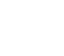# Thread: "0.9999..." = 1

1. ##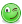"0.9999..." = 1

Report bad ads?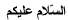I hope this hasn't been posted before. I just wondered how many of you know that the decimal number 0.9999...(recurring) actually equals 1.

Allow me to prove it for you:
Let
x = 0.9999...
Multiply both sides by ten:
10x = 9.9999...
Subtract x from both sides:
10x - x = 9.9999... - 0.9999...
9x = 9.0000...
Divide by nine:
x = 1.0000...
Anybody want to challenge me on this? Or perhaps somebody would like to post a different proof or counter-proof?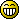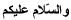2. ## Re: "0.9999..." = 1

I think my math teacher told me bout this...

i remember it from LI tho....straaange...

Oooh yehH! lool i was on LI while he was talking! :X hehe

3. ## Re: "0.9999..." = 1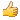woow gr8 mashallah.... who can challenge uand i dont no any other proof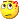4. ## Re: "0.9999..." = 1Hmm, seems interesting!, Barakalahu feek bro5. ## Re: "0.9999..." = 1I hope this hasn't been posted before. I just wondered how many of you know of the fact that the decimal number 0.9999...(recurring) actually equals 1.

Allow me to prove it for you:
Let
x = 0.9999...
Multiply both sides by ten:
10x = 9.9999...
Subtract x from both sides:
10x - x = 9.9999... - 0.9999...
9x = 9.0000...
Divide by nine:
x = 1.0000...
Anybody want to challenge me on this? Or perhaps somebody would like to post a different proof or counter-proof?Let
x = 0.9999...
Multiply both sides by ten:
10x = 9.9999...
Subtract x from both sides:

10x - x = 9.9999... - 0.9999... <-- You subtracted x on one side, and .999 on the other so it doesn't make sense.

Since 0.3333 + 0.3333 ) 0.3333.. = 0.999..
and since 0.333.. is 1/3, then 1/3 + 1/3 + 1/3 = 1

6. ## Re: "0.9999..." = 1

Let
x = 0.9999...
Multiply both sides by ten:
10x = 9.9999...
Subtract x from both sides:

10x - x = 9.9999... - 0.9999... <-- You subtracted x on one side, and .999 on the other so it doesn't make sense.
I don't see why it doesn't make sense, since x = .999...
The same thing is still being subtracted from both sides.

Since 0.3333 + 0.3333 ) 0.3333.. = 0.999..
and since 0.333.. is 1/3, then 1/3 + 1/3 + 1/3 = 1
Good one! Of course, that's provided that 1/3 does actually equal 0.333....

Regards

7. ## Re: "0.9999..." = 1

I don't see why it doesn't make sense, since x = .999...
The same thing is still being subtracted from both sides.

Good one! Of course, that's provided that 1/3 does actually equal 0.333....

Regards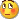Yes I was totally wrong. 1/3 does equal 0.33333333333333 : ).

8. ## Re: "0.9999..." = 1

1/3 does equal 0.33333333333333 : ).
Why? Because your Maths teacher told you?9. ## Re: "0.9999..." = 1

Why? Because your Maths teacher told you?No, my trusty calculator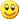10. ## Re: "0.9999..." = 1

No, my trusty calculatorOh.Sure, but aren't calculators programmed by us not-so trusty humans anyway?New to me.

12. ## Re: "0.9999..." = 1

Assalamu Alaykum

Me too^

How about 1 - 0.999999 = 0.000001

So 1 is not equal to 0.99999WaAlaykumus Salaam Warahmatullah!

13. ## Re: "0.9999..." = 1I hope this hasn't been posted before. I just wondered how many of you know of the fact that the decimal number 0.9999...(recurring) actually equals 1.

Allow me to prove it for you:
Let
x = 0.9999...
Multiply both sides by ten:
10x = 9.9999...
Subtract x from both sides:
10x - x = 9.9999... - 0.9999...
9x = 9.0000...
Divide by nine:
x = 1.0000...
Anybody want to challenge me on this? Or perhaps somebody would like to post a different proof or counter-proof?If x = 9, 9x does not = 9. If x = 9, 9x = 81.14. ## Re: "0.9999..." = 1

If x = 9, 9x does not = 9. If x = 9, 9x = 81.Wha..?15. ## Re: "0.9999..." = 1

The problem is the surrealism of infinity. In the real world where infinity doesn't exist, then in step 3 where you subtract x, there you'll see that the 9.999... always has one decimal less behind the point than 0.9999...
That way the subtractions can never become 9. So the calculations themself are fine, it's the mathematical axiom of infinity that is dodgy.

The same happens for 1/3 = 0.333... that only works with an infinite number of factors. In reality it's impossible to calculate with that, so we calculate in approximations (so does your calculator).

16. ## Re: "0.9999..." = 1How about 1 - 0.999999 = 0.000001

So 1 is not equal to 0.99999Yes, but remember that the 0.999... is a recurring decimal with an infinite number of nines. For this reason, 1 - 0.9999.... would result in 0.0000000......

There would be an infinite number of zero's in the answer so there would be no 1 at the end because there is no end!17. ## Re: "0.9999..." = 1If x = 9, 9x does not = 9. If x = 9, 9x = 81.That certainly makes sense, but the argument here is that 0.9999... = 1, where x = 0.99999...

Nobody claimed that x = 9!18. ## Re: "0.9999..." = 1

To clarify the previous post, consider the same calculation, only this time with finite numbers instead of infinite numbers:

x = 0.9999
Multiply both sides by ten:
10x = 9.999
Subtract x from both sides:
10x - x = 9.999 - 0.9999
9x = 8.9991
Divide by nine:
x = 0.9999

19. ## Re: "0.9999..." = 1Subtract x from both sides:
10x - x = 9.9999... - 0.9999...
9x = 9.0000...
How come 10x - x becomes 9x, when x=0.9999...?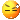20. ## Re: "0.9999..." = 1I hope this hasn't been posted before. I just wondered how many of you know of the fact that the decimal number 0.9999...(recurring) actually equals 1.

Allow me to prove it for you:
Let
x = 0.9999...
Multiply both sides by ten:
10x = 9.9999...
Subtract x from both sides:
10x - x = 9.9999... - 0.9999...
9x = 9.0000...
Divide by nine:
x = 1.0000...
Anybody want to challenge me on this? Or perhaps somebody would like to post a different proof or counter-proof?Who ever showed you that needs to get a good smack in the head.

I suggest you go to a good math teacher and allow them to properly educate you on the rules of mathematics.

Your "equation" boils down to ((x * 10) - x)/9 = something

You've allowed x to be an irrational number, which is fine, but that's where you are having difficulties. Logically speaking, ((x * 10) - x)/9 should equal x again.

Try .888888888 and see what you get with your logic.

(0.888888888..... * 10 - 0.888888888..... )/8 = ?
(8.888888888..... - 0.888888888..... )/8 = What do you think it should be?

Please don't make a mockery of mathematics.

All the best wishes,

Faysal

21.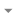Hide
Page 1 of 2 1 2 Last
Hey there!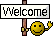Looks like you're enjoying the discussion, but you're not signed up for an account.

When you create an account, we remember exactly what you've read, so you always come right back where you left off. You also get notifications, here and via email, whenever new posts are made. And you can like posts and share your thoughts.Sign Up

####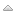Posting Permissions

• You may not post new threads
• You may not post replies
• You may not post attachments
• You may not edit your posts
•
create